# NCERT Solutions For Class 6 Maths Chapter 13 Symmetry

NCERT Solutions For Class 6 Maths Chapter 13 Symmetry

### NCERT Solutions For Class 6 Maths Chapter 13 Symmetry Ex 13.1

NCERT Solutions For Class 6 Maths Chapter 13 Symmetry Ex 13.1

Exercise 13.1

Ex 13.1 Class 6 Maths Question 1.
List any four symmetrical objects from your home or school.
Solution:
The following objects can be symmetrical:
(a) Notebook
(b) Dinning table
(c) A blackboard
(d) Wall clock
(e) A pair of scissors

Ex 13.1 Class 6 Maths Question 2.
For the given figure, which one is the mirror line, l1 or l2?
Solution:
In the following figure, l2 is the mirror line.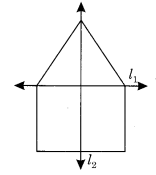Ex 13.1 Class 6 Maths Question 3.
Identify the shapes given below. Check whether they are symmetrical or not. Draw the line of symmetry as well.Solution:
(a) The given symmetric figure is a lock in which vertical line ‘l’ is the line of symmetry.(b) The given figure is a symmetrical bucket in which vertical line ‘l’ is the line of symmetry.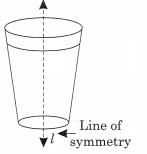(c) The given figure is not symmetrical.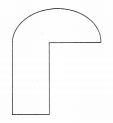(d) The given figure is a symmetric telephone in which vertical line l is called the line of symmetry.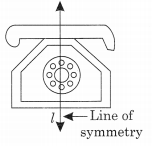(e) The given figure is symmetrical. Horizontal line l is called the line of symmetry.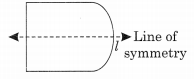(f) The given figure is symmetrical. Vertical line l is called its line of symmetry.Ex 13.1 Class 6 Maths Question 4.
Copy the following on a squared paper. A square paper is what you would have used in your arithmetic notebook is earlier classes. Then complete them such that the dotted line is the line of symmetry.Solution: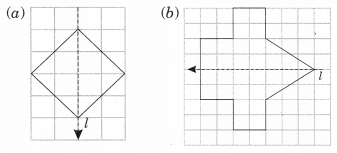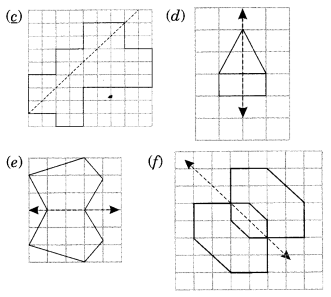Ex 13.1 Class 6 Maths Question 5.
In the figure, l is the line of symmetry. Complete the diagram to make it symmetric.Solution:
The completed figure is as follows: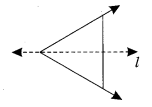Ex 13.1 Class 6 Maths Question 6.
In the figure, l is the line of symmetry. Draw the image of the triangle and complete the diagram so that it becomes symmetric.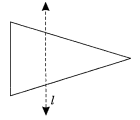Solution:
The symmetric figure is given as follows.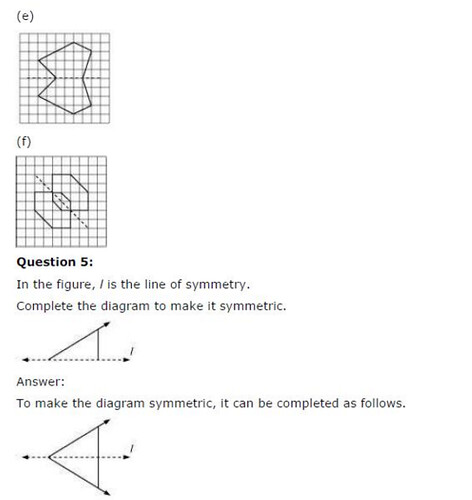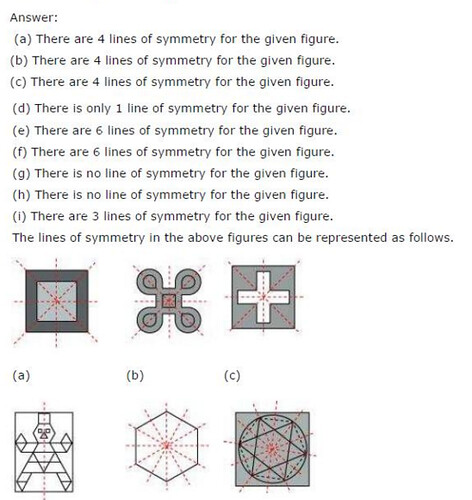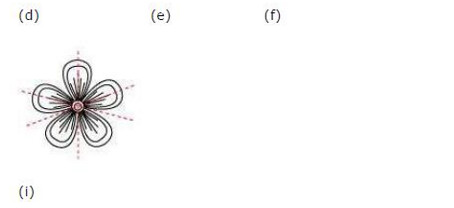JEE Main Application Fee

 JEE Main Application Fees For Centres in India General/OBC ST/SC/PWD Either Paper I or Paper II Boys Rs. 1000 Rs. 500 Girls Rs. 500 Rs. 500 Both Paper I or Paper II Boys Rs. 1800 Rs. 900 Girls Rs. 900 Rs. 900 JEE Main Application Fees For Centres in Foreign Country General/OBC ST/SC/PWD Either Paper I or Paper II Boys Rs. 2500 Rs. 1250 Girls Rs. 1250 Rs. 1250 Both Paper I or Paper II Boys Rs. 3800 Rs. 1900 Girls Rs. 1900 Rs. 1900

## SabDekho

The Complete Educational Website